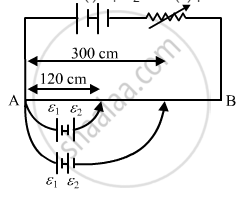Share

# Figure Shows a Long Potentiometer Wire Ab Having a Constant Potential Gradient. the Null Points for the Two Primary Cells of Emfs ε1 And ε2 Connected in the Manner Shown Are Obtained at a - Physics

ConceptPotentiometer

#### Question

Figure shows a long potentiometer wire AB having a constant potential gradient. The null points for the two primary cells of emfs ε1 and ε2 connected in the manner shown are obtained at a distance of l1 = 120 cm and l2 = 300 cm from the end A. Determine (i) ε12 and (ii) position of null point for the cell ε1 only.#### Solution

(i)  Let x be the resistance per unit length of the potentiometer wire and I be the constant current flowing through it. Then from the figure, we have:

$\epsilon_1 - \epsilon_2 = \left( 120x \right)I . . . (1)$ and
$\epsilon_1 + \epsilon_2 = \left( 300x \right)I . . . (2)$
Dividing both equations, we get:

$\frac{\epsilon_1 - \epsilon_2}{\epsilon_1 + \epsilon_2} = \frac{120}{300}$

$\Rightarrow 180 \epsilon_1 = 420 \epsilon_2$

$\Rightarrow \frac{\epsilon_1}{\epsilon_2} = \frac{7}{3}$

(ii) Now adding equations (1) and (2), we get:

$2 \epsilon_1 = \left( 420x \right)I$

$\Rightarrow \epsilon_1 = \left( 210x \right)I$

Comparing with

$\epsilon = \left( Lx \right)I$we get:
length of balancing point, L = 210 cm for cell 1

Is there an error in this question or solution?

#### APPEARS IN

Solution Figure Shows a Long Potentiometer Wire Ab Having a Constant Potential Gradient. the Null Points for the Two Primary Cells of Emfs ε1 And ε2 Connected in the Manner Shown Are Obtained at a Concept: Potentiometer.
S